• RU
•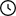Waiting For Moderation: 26

# Analysis of kinematic mechanism of lever

• Size: 2 MB

## Description

Coursewoman in the discipline 'Theory of machines and mechanisms'. In this folder there are drawings: 1) Kinematic analysis of the lever mechanism, 2) Power analysis of the lever mechanism, 3) Synthesis and analysis of the gear mechanism, 4) Synthesis and analysis of the cam mechanism, 5) Calculation of the flywheel. Also attached is an explanatory note with all calculations

## Project's Contentteorija_mashin_i_mehanizmov.rar [ 2 MB ]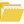курсовик по тмм1.dwg [ 138 KB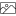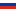]2.dwg [ 124 KB]3.dwg [ 97 KB]4.dwg [ 122 KB]5.dwg [ 147 KB]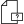plot.log [ 2 KB ]Книга1.xlsx [ 68 KB ]ПЗ(сод).docx [ 31 KB]поясн записка.docx [ 1 MB]3.bak [ 108 KB ]

### 4 Synthesis and analysis of cam mechanism

In general, the task of synthesizing the cam mechanism is to select the kinematic scheme and the law of motion of the pusher, to provide the minimum dimensions of the mechanism taking into account dynamic indicators (permissible pressure angles, permissible increase factors of forces, etc.) and to determine the geometric shape of the cam profile.

When calculating cam mechanisms, it is necessary to solve the following problems:

1. Determine the time of individual phases;

2. Determine the values of movements, speeds and accelerations of the pusher and construct their diagrams;

3. Determine the minimum radius of the theoretical cam profile; if it is specified, compare it with the one found by calculation; Define other mechanism parameters that are not specified.

4. Build theoretical and practical cam profiles and show transmission angles or pressure angles.

### 4.1 Initial data of the task

Far stop phase -

Return Phase -

Maximum pusher movement

Rotation direction - counterclockwise

Allowable pressure angle

Mechanism kinematic diagram - cam mechanism with translational pusher

The law of changing the acceleration of the pusher is rectangular.

### 4.4 Cam profiling

When you graphically draw a cam profile, it is best to use the motion reversal method. And for mechanisms with a flat pusher, this method is the only possible.

The method of motion reversal consists in the fact that all links of the cam mechanism, including the post, are conventionally informed by rotation around the center of the cam at an angular speed equal to the angular speed of the cam, but directed in the opposite direction. The relative motion of the links of the mechanism is maintained, and the angular velocity of each link in the reversed motion will be equal to the algebraic sum of the angular velocity of the real motion and (¼ 1). For example, if the cam had an angular velocity (1) and add an angular velocity (1) to it, the cam becomes as if stationary, that is, turns into a fixed profile curve. The post receives rotation at angular speed (¼ 1), and the condition of touching the pusher with the cam makes it possible to determine in sequence a number of points of the cam profile. If you connect these points to a smooth curve, you get the full cam profile.

The source data you need to create a cam profile:

Initial data: cam mechanism diagram, initial cam radius rn = 134.8mm, eccentricity e = 20mm, pusher movement graph.

### 4.5.1. To Create a Center Profile

When constructing the cam profile, all linear values ​ ​ are deposited in the same scale μ_ s = 1.6875 m/mm.

Build Order:

1. A circle with the center O1 is carried out, the radius of which is equal to the initial radius of the cam rn = 134.8 mm.

2. Concentric circle is drawn from the same center O1 with radius equal to eccentricity.

3. Denotes the direction of rotation of the cam, which is 1.

4. With respect to circle e, a line parallel to the pusher path is drawn on the side where the linear velocity of the touch point as the material point of the future cam coincides in the direction with the vector of the pusher speed in the removal phase (point 0 - zero). This arrangement reduces the pressure angles in the removal phase.

5. The circle of radius e is divided into as many equal parts as the abscissa axis of the motion graph has. The points are numbered in the direction opposite to the direction of rotation of the cam (reversed motion).

6. Trajectories of pusher motion in facing motion are passed through obtained points (2,4,6, etc.) with respect to circle e.

7. From points 2.4.6 etc. of intersection of trajectories with the circle of the initial radius, segments 22. 4-4. 66 etc. corresponding to segments 22. 4-4. 6-6 etc. of the motion graph are deposited.

8. Points 2,4,6 etc. are connected by a smooth curve, which will be the center profile of the cam.

9. Phase angles (by cam) are marked according to movement schedule

φ at, φд, oh, φв and φб, about between straight lines of O10, O 120, O 124 and O 10, way of their postponement it is consecutive against rotation of a cam. Forward O1 0 is taken as the starting point.

### 4.5.2 Determination of roller radius

To avoid sharpening the cam profile, the radius of the roller must be less than the minimum curvature radius of the center profile. The lowest contact stresses between the roller and the cam structural profile for the convex portion thereof will occur with the following roller dimensions:

rρ= ( 0,65…0,8)ρmin ,

where αmin is the smallest radius of curvature of the center profile. The value of αmin is defined as follows: the point B is determined on the eye, at which the radius of curvature of the center profile seems to be the smallest. Near this point, on either side of it, two more points B and B are selected, which are connected to point B by chords. Each chord is divided into two equal parts and perpendicular to chordes are passed through the obtained points. The point O of intersection of the perpendicular points, equal to the points B, B and B, will be the center of curvature of the intended section of the profile.

ρmin = OB = OB = OB=85,34 мм.

However, often it turns out to be quite large (commensurate with the initial radius). In this case, the radius of the roller is calculated by the formula:

rp ≤ 0, 4rn = 0, 4· 134.8 = 53.92 mm. Therefore, finally the radius of the roller is taken equal to the smallest value calculated according to two formulas:

rp = 53.92mm

### 4.5.3 Construction of structural profile

From points 2, 4, 6, etc. of the center profile, as from the centers, a number of circles are drawn with a radius equal to the radius of the roller. The inner envelope to the successive positions of the roller will be the structural profile of the cam. In the drawing, the envelope is a bold line, the center profile is a thin line.

### 5 Flywheel calculation

Define flywheel dimensions for the mechanism. Motion unevenness coefficient 1 = 0.05, average angular velocity of driving link sr = 1 = 18 s-1.

Regardless of the method used for calculating the flywheel, it is first necessary to construct diagrams of the reduced moment of inertia Jp and the reduced moment of resistance Ms as a function of the angle of rotation of the crank.

Drawings content1.dwg2.dwg3.dwg4.dwg5.dwg